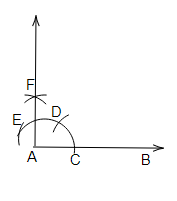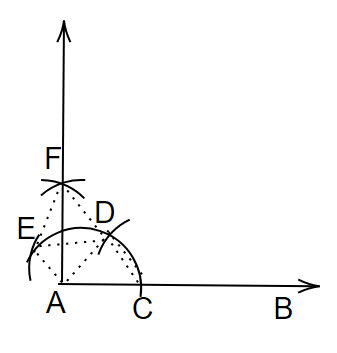# Construct an angle of $90^{\circ}$ at the initial point of a given ray and justify the construction.

To do:

We have to construct an angle of $90^o$ at the initial point of a given ray and justify the construction.

Solution:Steps of construction:

(a) Draw a ray $AB$.

(b) With centre $A$ and a suitable radius, draw an arc such that it cuts $AB$ at $C$.

(c) With centre $C$ and the same radius taken above cut the above arc at $D$.

(d) With centre $D$ and the same radius taken above cut the above arc at $E$.

(c) With $D$ and $E$ as centers and radius more than $\frac{1}{2}DE$ draw two arcs meeting each other at $F$.

(d) Join $AF$ and produce it to form ray $AF$.

Therefore,

$\angle BAF = 90^o$.

Justification:

To justify $\angle BAF=90^o$

Let us draw an imaginary line from $A$ to $D$ and $O$ to $E$.We have,

$AC=CD=AD$

Therefore,

$ACD$ is an equilateral triangle

This implies,

$\angle CAD=60^o$

In a similar way, we get,

$AE=DE=AD$

Therefore,

$ADE$ is an equilateral triangle

This implies,

$\angle EAD=60^o$

By SSS congruence rule we get,

$\triangle ACD \cong \triangle ADE$

From C.P.C.T We get,

$\angle CAD=\angle EAD$

Therefore,

$\angle DAF=\frac{1}{2}EAD=\frac{1}{2}(60^o)=30^o$

This implies,

$\angle DAF=30^o$

$\angle BAF=\angle BAD+\angle DAF$

$=60^o+30^o$

$=90^o$

Therefore, justified.

Updated on: 10-Oct-2022

25 Views